# Isothermal Carbonization of Wood Particle: Application of the Lattice Boltzmann Method

Isothermal Carbonization of Wood Particle: Application of the Lattice Boltzmann Method

Ahmed Mahmoudi Imen Mejri

Unité de Recherche Matériaux, Energie et Energies Renouvelables (MEER), Faculté des Sciences de Gafsa, B.P.19, Zarroug, Gafsa, 2112, Tunisie

Corresponding Author Email:
ahmed.mahmoudi@yahoo.fr
Page:
129-134
|
DOI:
https://doi.org/10.18280/ijht.330221
N/A
|
Accepted:
N/A
|
Published:
30 June 2015
| Citation

OPEN ACCESS

Abstract:

In this paper, the lattice Boltzmann method (LBM) is proposed as a potential solver for one-dimensional heat and mass transfer for isothermal carbonization of wood particles. To check the validity of this method, the LBM results are compared with the published résults and a good agreement is obtained. The LBM model is also tested for different operating conditions (reactor temperature and particle size) and for different types of wood particle to study the evolution of the local temperature and mass loss inside the wood particle.

Keywords:

Lattice Boltzmann method, Carbonization, Wood particle, Conduction, Planar medium.

1. Introduction

Wood carbonization is a promising route for production of charcoal as well as gaseous fuels. However, experimental results show that the thermal decomposition of the material wood is an unpredictable and complex process. Particularly, the complexity of some phenomena during the carbonization of thick wood particles, such as heat and mass transfer within the wood, chemical reactions and mechanical deformations, and the absence of detailed information about the chemical kinetics and the evolution of the thermal properties of wood in the different stages of decomposition from the virgin wood up to the charcoal constitute a major problem to the development of relevant models of carbonization. Salazar et al. – studied the influence of the size and shape of the wood particles on the pyrolysis mechanisms. According to these authors, the thermal decomposition of wood results in three parallel reactions of first order, corresponding respectively to the decomposition of hemicellulose, cellulose and lignin. The reactions rate is described by an Arrhenius formalism, whose kinetic parameters are determined experimentally. This model gave satisfaction only for wood particles whose diameters are lower than 20mm. Koufopanos et al.  defined a model in which they coupled conduction heat transfer with chemical kinetics. They concluded that the reactions heat can be represented by endothermic or exothermic values according to the conversion rate. The model predictions show a good agreement with experimental data relevant to a diameter of 20mm. Di Blasi and Russo ,  proposed a model based on three primary reactions and two secondary reactions that

follow the formalism of Arrhenius. Also, the model takes into account conduction, convection and radiation heat transfer. The validation of this model with the experimental data of Lee et al.  relevant to a diameter of 25mm shows good predictions only for short reaction times. Gronli  developed a transient mono-dimensional model for the simulation of humid wood drying and pyrolysis. This model is based on a wood decomposition scheme including three parallel reactions leading to simultaneous production of gas, tar and charcoal and takes into account interactions between mass, momentum and heat transfer in the porous structure of the wood. The model leads to the study of the effect of the wood particle diameter (1≤d≤100mm) and the intensity of the heating flux (50≤Ф≤200kWm²) on the temperature profile and the production rate of different products. The predictions of this model are in good agreement with their experimental data. Larfeldt et al.  have modified the mono-dimensional model of Melaaen  to take into account the structural changes during pyrolysis of the wood. In comparison with the experimental data, the results of the modified model show an important reduction of pyrolysis time. Peters and Bruch  have developed a numerical study similar to that of Gronli  that was positively validated experimentally. Abbassi et al.  investigated into pyrolysis of biomass both experimentally and numerically. The reactor was divided into three zones that were treated as perfectly stirred reactors. Tar as a major product of pyrolysis was assumed to crack into methane CH4, carbon monoxide CO, carbon dioxide CO2 and hydrogen H2, determined by an Arrhenius expression. The model was validated with measurements and was used to control plant operation. Rattea et al.  predicted pyrolysis of wood waste by a model approach developed earlier by Peters  and proved that this approach may also be employed to large wood particles. The latter approach has also been used by Sadhukhan et al.  for large wood particles and they emphasised that intra-particle convection is important. Dupont et al.  performed biomass pyrolysis also in an entrained flow reactor under high temperatures from 1073 to 1273K and high heat fluxes (10–100kW/m2). Their experimental results were compared to predictions of the kinetic mechanism of Ranzi et al.  and encouraged design and understanding of industrial gasifiers.

The thermal lattice Boltzmann method has been widely used in many kinds of complex flows such as solar collectors, multiphase flow and micro flow. Mejri et al.  studied 1-D conduction-radiation problem by lattice Boltzmann method. LBM is used to solve the energy equation and the radiative transfer equation. The found results are compared with those available in the literature and a very good agreement was found. Mahmoudi et al.  studied the effect of the magnetic field intensity and direction on natural convection in a square enclosure filled with nanofluid. The found results show that the heat transfer and fluid flow depends strongly upon the direction of magnetic field. Mejri et al.  studied magnetic field effect on natural convection in a nanofluid filled enclosure with non-uniform heating on both side walls.

The aim of this work is to apply the LBM to predict heat and mass transfer during the carbonization of wood particle. Which to the knowledge of the authors, is applied at the first time to carbonization of wood particle. The proposed model takes into account the heat and mass transfer and chemical kinetics simultaneously. The thermal properties of wood are considered to be linear functions of the local temperature and have been estimated from literature data. The carbonization process has been described by a kinetic scheme based on a two stage semi-global kinetic model. The model is used to study the effect of reactor temperature,particle size and wood particule type on the evolution of the local temperature and mass loss inside the wood particle.

2. Mathematical Formulation

2.1 Problem statement

Fig.1 shows the physical model for the considered problem, a cylindrical dried wood particle is placed in a reactor at constant temperature (TR). The heat transfer at the particle surface takes place essentially by convection and radiation. As the temperature increases at the surface, heat diffuses inside the wood by conduction. The following carbonization reaction scheme, developed recently by the authors , is used here:

A1(1st pseudo component of wood)→G1(gaz)         (1)

A2(2nd pseudo component of wood)→γ2C2(char)+G2(gaz)       (2)

A3(3rd pseudo component of wood)→βB(intermediate solid)+G3(gaz)    (3)

B(intermediate solid)→ γ3C3(char)+G4(gaz)          (4)

## 1.pngFigure 1. Physical model of isothermal carbonization of a cylindrical wood particle

By assuming that the kinetics is described by the first order laws of Arrhenius for the four reactions, the mass balance of the solid and slightly volatile components A1, A2, A3, C2, B and C3, can be written, respectively:

$\frac{\partial \rho_{\mathrm{A}_{1}}}{\partial t}=-k_{1} \rho_{\mathrm{A}_{1}}$     (5)

$\frac{\partial \rho_{\mathrm{A}_{2}}}{\partial t}=-k_{2} \rho_{\mathrm{A}_{2}}$   (6)

$\frac{\partial \rho_{A_{3}}}{\partial t}=-k_{3} \rho_{\mathrm{A}_{3}}$    (7)

$\frac{\partial \rho_{\mathrm{C}_{2}}}{\partial t}=\gamma_{2} k_{2} \rho_{\mathrm{A}_{2}}$   (8)

$\frac{\partial \rho_{\mathrm{B}}}{\partial t}=\beta k_{3} \rho_{\mathrm{A}_{3}}-k_{4} \rho_{\mathrm{B}}$    (9)

$\frac{\partial \rho_{\mathrm{C}_{3}}}{\partial t}=\gamma_{3} k_{4} \rho_{\mathrm{B}}$    (10)

where

$k_{i}=k_{0 i} \exp \left(E_{a i} / R T\right)$ for $i=1,2,3$ and 4    (11)

The activation energies $E_{a i}$ and pre-exponential coefficients $k_{0 i}$ are given in Table.1 and $\gamma_{2}$ , $\beta$  and $\gamma_{3}$ are defined by experimental correlations in .

Table 1. Kinetics data of wood carbonization 

 Reaction rate$k_{i}\left(s^{-1}\right)$ Activation energy $E_{a i}\left(\mathrm{kJmol}^{-1}\right)$ Pre-exponential constant $k_{0 i}\left(s^{-1}\right)$ $k_{1}$ 105.89 $3.5 \times 10^{7}$ $k_{2}$ 106.78 $3.72 \times 10^{6}$ $k_{3}$ 169.56 $7.23 \times 10^{11}$ $k_{4}$ 51.04 $3.4 \times 10^{-1}$

2.2 Energy equation

The energy equation for a control volume during carbonization is:

$\rho c_{p w} \frac{\partial T}{\partial t}=\vec{\nabla} \cdot\left(\lambda_{w} \vec{\nabla} T\right)+S$     (12)

$S=\left(k_{1} \rho_{\mathrm{A}_{1}}+k_{2} \rho_{\mathrm{A}_{2}}+k_{3} \rho_{\mathrm{A}_{3}}\right) \Delta H+k_{4} \rho_{\mathrm{B}} \Delta H_{4}$    (13)

$\rho=v_{1} \rho_{\mathrm{A}_{1}}+v_{2}\left(\rho_{\mathrm{A}_{2}}+\rho_{C_{2}}\right)+v_{3}\left(\rho_{\mathrm{A}_{3}}+\rho_{\mathrm{B}}+\rho_{\mathrm{C}_{3}}\right)$   (14)

Where S is the power generated by the exothermic reaction of the wood decomposition,$\rho$ is the wood density and $V_{i=1,2,3}$ are the mass fraction, its values are reported in . Thermo-physical properties of wood vary linearly with the local temperature and are estimated from relations published in the literature. These relations are given in Table.2.

For the resolution of Eq. (12), the following boundary conditions are considered:

At particle axis: $\left.\frac{\partial T}{\partial r}\right|_{r=0}=0$    (15)

At the surface:

$\left.\frac{\partial T}{\partial r}\right|_{r=d / 2}=-\frac{h}{\lambda_{w}}\left(T_{R}-T(r=d / 2, t)\right)$

Where h represents the global heat transfer coefficient these values are reported in Table.3. The mass and energy conservation equations constitute a coupled nonlinear partial differential equations system that can be solved simultaneously by the LBM.

Table 2. Thermo-physical Properties of Wood 

 Thermal conductivity of wood $\left(\mathrm{Wm}^{-1} \mathrm{K}^{-1}\right)$ $\lambda_{w}=0.13+3 \times 10^{-4} \times(T-273)$ Specific heat of wood $\left(\mathrm{JKg}^{-1} \mathrm{K}^{-1}\right)$ $c_{p w}=1112+4.85 \times(T-273)$ Heat of reaction (1,2,3) $\left(\mathrm{JKg}^{-1}\right)$ $\Delta H_{4}=42 \times 10^{3}$ Heat of reaction (4) $\left(\mathrm{JKg}^{-1}\right)$ $\Delta H=100 \times 10^{3}$

Table 3. Global heat transfer coefficient for different reactor temperatures 

 Reactor temperature TR (K) Global heat transfer coefficient $\left(\mathrm{Wm}^{-2} \mathrm{K}^{-1}\right)$ 623 50 673 61

2.3 Lattice Boltzmann Simulation

The discrete Boltzmann Equation with Bhatanagar-Gross-Krook (BGK) approximation is given by :

$\frac{\partial f_{i}(\vec{r}, t)}{\partial t}+\vec{e}_{i} \cdot \nabla f_{i}(\vec{r}, t)=-\frac{1}{\tau}\left[f_{i}(\vec{r}, t)-f_{i}^{e q}(\vec{r}, t)\right] \quad i=1 \ldots m$  (16)

Where $f_{i}$ is the particle distribution function denoting the number of particles at the lattice node $\vec{r}$ and at the time t, moving in the direction i with the velocity $\vec{e}_{i}$ along the lattice $\Delta r=e_{i} \Delta t$ connecting the neighbors, m is the number of directions, $\tau$ is the relaxation time, and $f^{e q}_{i}$ is the equilibrium distribution function.

After discretization, (Eq.15) is written as:

$f_{i}\left(\vec{r}+\vec{e}_{i} \Delta t, t+\Delta t\right)=f_{i}(\vec{r}, t)-\frac{1}{\tau}\left[f_{i}(\vec{r}, t)-f_{i}^{e q}(\vec{r}, t)\right]$       (17)

The temperature is obtained after summing $f_{i}$ overall direction:

$\mathrm{T}(\vec{r}, t)=\sum_{i=1, m} f_{i}(\vec{r}, t)$    (18)

To process Eq. (17), an equilibrium distribution function is required, which for the considered problem is given by:

$f_{i}^{e q}(\vec{r}, t)=\mathbf{w}_{i} \mathbf{T}(\vec{r}, t)$   (19)

To account for the source term, the energy equation in the LBM formulation, Eq. (17) is modified to:

$f_{i}\left(\vec{r}+\vec{e}_{i} \Delta t, t+\Delta t\right)=f_{i}(\vec{r}, t)-\frac{1}{\tau}\left[f_{i}(\vec{r}, t)-f_{i}^{e q}(\vec{r}, t)\right] -\frac{\Delta t \mathrm{w}_{i}}{\rho(\vec{r}, t) c_{P w}(\vec{r}, t)} S(\vec{r}, t)$        (20)

The relaxation time $\tau$ for the D1Q2 lattice is computed from:

$\tau=\frac{\lambda_{w}}{\left|\vec{e}_{i}\right|^{2} \rho c_{p w}}+\frac{\Delta t}{2}$     (21)

For this lattice, the two velocities $e_{1}$ and $e_{2}$ , and their corresponding weights $\mathrm{W}_{1}$ and $\mathbf{W}_{2}$ are given by:

$e_{1}=\frac{\Delta r}{\Delta t} \quad e_{2}=-\frac{\Delta r}{\Delta t}$      (22)

$\mathrm{w}_{1}=\mathrm{w}_{2}=\frac{1}{2}$    (23)

3. Results and Discussion

In order to check on the accuracy of the numerical technique employed for the solution of the considered problem, the present numerical code was validated with the published experimental results (Fig.2a-c) of Koufopanos et al.  for isothermal carbonization of a cylindrical wood particle of $d=20 \times 10^{-3} \mathrm{m}$ diameter and $\rho_{\mathrm{w}}=650 \mathrm{kg} \mathrm{m}^{-3}$ density at reactor temperatures of TR = 623 and 673 K.

Fig.2a shows the local temperature evolution at the axis of the wood particle at reactor temperature TR = 623 K. the results show that the model describes correctly the temperature profile at the axis of the wood particle. Fig.2b shows the local temperature evolution at $r=d / 4$ at reactor temperature of 673 K. An excellent agreement is found. Fig.2c shows the residual density evolution of the wood particle. An excellent agreement is also found.

The present LBM algorithm is also validated with the numerical results of Grioui et al  (Fig.3a-c) obtained by the finite difference method using an implicit scheme, for isothermal carbonization of a cylindrical olive wood particle of $d=20 \times 10^{-3} \mathrm{m}$ diameter and $\rho_{\mathrm{w}}=879 \mathrm{kg} \mathrm{m}^{-3}$ density at reactor temperatures of TR = 623K.

Fig.3a shows the local temperature evolution at the surface of the wood particle. Fig.3b and c show the local residual density evolution respectively at the axis and at the surface of the wood particle. Fig.3a-c shows that the LBM numerical results are in good agreement with the numerical results obtained by Grioui et al .

## 2a.png## 2b.png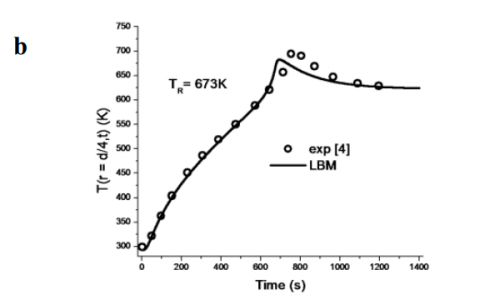## 2c.png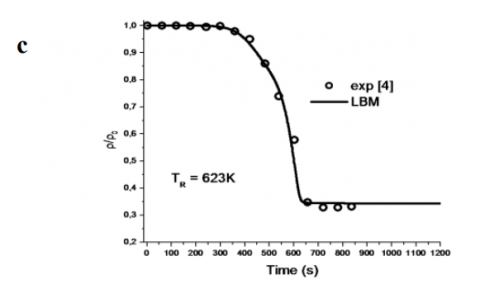Figure 2. Validation of the LBM model with the experimental results of Koufopanos et al. .

## 3a.png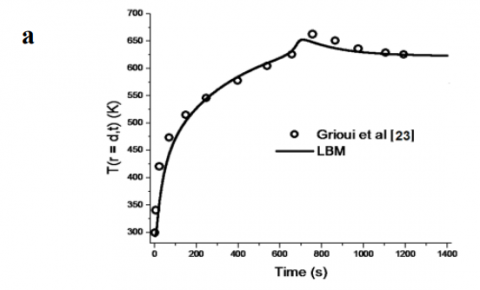## 3b.png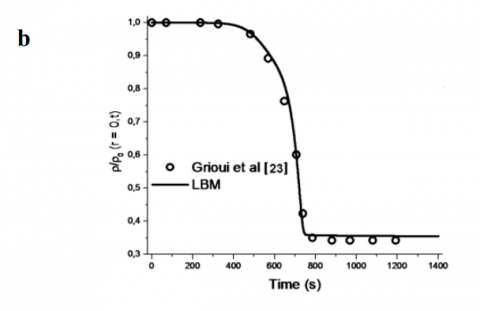## 3c.png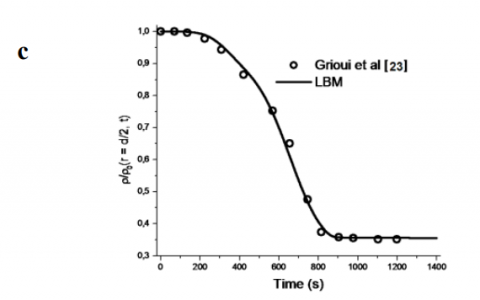Figure 3. Validation of the LBM model with the numerical results of Grioui et al. 

Based on the aforementioned comparisons, the developed Model is reliable for studying wood particle carbonization. Subsequently the LBM model is tested for different operating conditions and different types of wood particle.

Fig.4a, b and c show respectively the temperature profile, the residual density and the density loss rate at the surface and inside of the olive wood particle $\left(\rho_{\mathrm{w}}=879 \mathrm{kg} \mathrm{m}^{-3}\right)$ during isothermal carbonization at TR = 623 K. The obtained results show that the LBM model predicts correctly the carbonization process inside the olive wood particle.

## 4a.png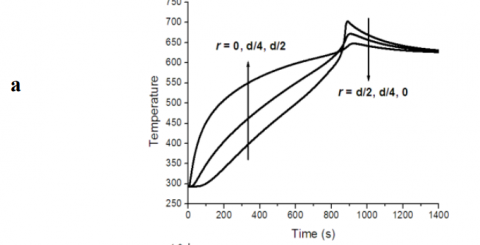## 4b.png## 4c.pngFigure 4. Evolution of the (a) local temperature (b) local residual density (c) local density loss rate inside an olive wood particle

Fig.5a, b and c show respectively the temperature profile, the residual density and the density loss rate during isothermal carbonization (TR = 623K), for different types of wood particle; fir($\rho_{\mathrm{w}}=435 \mathrm{kg} \mathrm{m}^{-3}$) , oak $\left(\rho_{w}=650 \mathrm{kg} \mathrm{m}^{-3}\right)$ and olive $\left(\rho_{\mathrm{w}}=879 \mathrm{kg} \mathrm{m}^{-3}\right)$ . The obtained results show that the LBM model predicts correctly the effect of the wood density on the carbonization process.

## 5a.png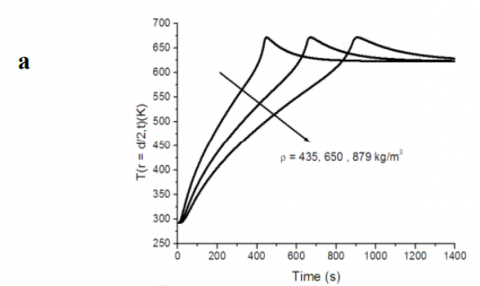## 5b.png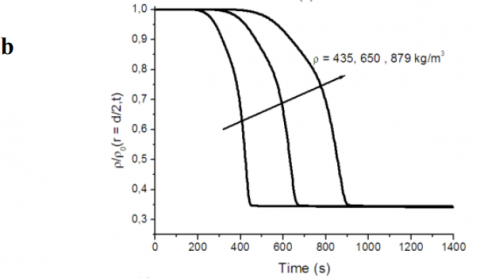## 5c.pngFigure 5. Evolution of the (a) local temperature (b) local residual density (c) local density loss rate for different types of wood particle

Fig.6 and 7 show respectively the effect of the reactor temperature TR and the wood particle size d on the evolution of the heat and mass transfer mechanisms during the carbonization of a cylindrical olive wood particle $\left(\rho_{\mathrm{w}}=879 \mathrm{kg} \mathrm{m}^{-3}\right)$ . The obtained results show that the developed algorithm predicts correctly the dependency of the carbonization process of the considered parameters.

## 6a.png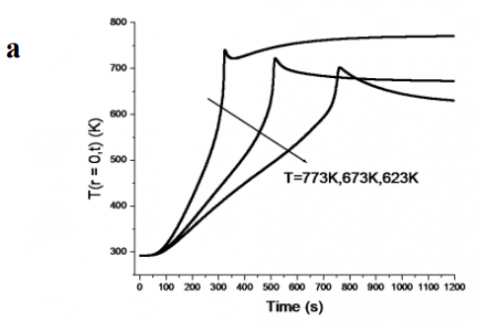## 6b.png## 6c.png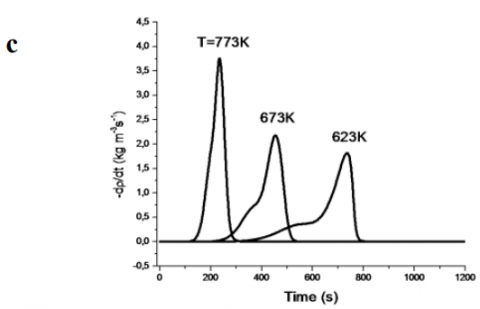Figure 6. Effect of the reactor temperature on the (a) local temperature (b) local residual density (c) local density loss rate

## 7a.png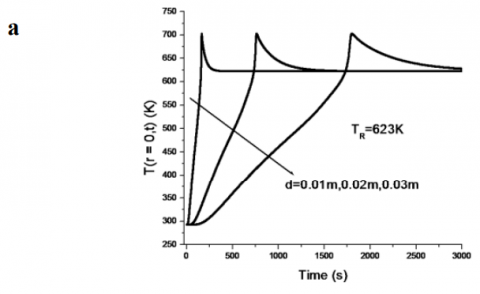## 7b.png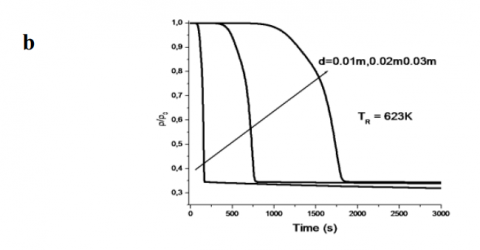## 7c.png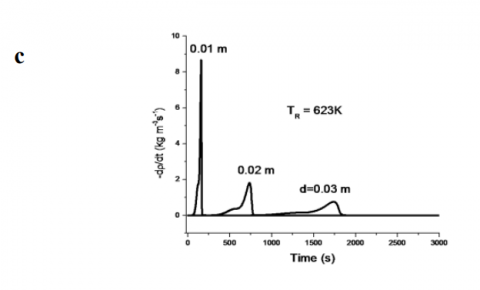Figure 7. Effect of the wood particle size on the (a) local temperature (b) local residual density (c) local density loss rate

4. Conclusions

A numerical simulation by the lattice Boltzmann method (LBM) is proposed for solving one-dimensional heat and mass transfer for isothermal carbonization of wood particles. Results of the carbonization of wood particle are obtained and compared to the published results. The good agreement between the lattice Boltzmann results and published results, also, the various tests performed on the model show that the proposed model is able to predict correctly the physical phenomena of carbonization.

Nomenclature
 cp Specific heat at constant pressure (JKg-1K-1) ei Lattice speed  (m/s) f Internal energy distribution functions (K) f eq Equilibrium internal energy distribution (K) h Global heat transfer coefficient (Wm-2K-1) t Time (s) T Temperature (K) w Weight in the LBM GREEK SYMBOLS Δr Lattice spacing (m) Δt Time increment (s) λ Thermal conductivity (Wm-1K-1) ρ Density (Kg/m3) τ Relaxation time (s) SUBSCRIPT R Reactor w Wood
References

1. M.A. Connor and C.M. Salazar. The pyrolysis of wood at low heating rates, In: Proc forest products research international achievements and the future. Carbon from biomass, pp. 5-12. 1985.

2. C.M .Salazar. PhD thesis, University of Melbourne, Australia, 1987.

3. M.A Connor and C.M Salazar. Factors influencing the decomposition processes in wood particles during low temperature pyrolysis, In: Bridgwater AV, Kuester JL, editors. Proc research in thermochemical biomass conversion. Londre, UK: Elsevier Applied Science Publisher, pp. 164–78, 1988.

4. C.A .Koufopanos, N. Papayannakos, G. Maschio and A. Lucchesi. Modelling of the pyrolysis of biomass particles. Studies on kinetics thermal and heat transfer effects, Can J Chem Eng, pp. 907–915. 1991.

5. C. Di Blasi and G. Russo. Modeling of transport phenomena and kinetics of biomass pyrolysis, In: Bridgwater AV, editor. Proc advances in thermochemical biomass conversion. Glasgow, UK: Blackie Academic & Professional Publishers. pp. 906–921, 1994.

6. C. Di Blasi. A transient, two dimensional model of biomass pyrolysis, In: Bridgwater AV, Boocock DGB, editors. Proc developments in thermochemical biomass conversion. Glasgow, UK: Blackie Academic & Professional Pub .pp.147–160, 1997.

7. C.K .Lee, R.F Chaiken and J.M. Singer. Charring pyrolysis of wood in fires by laser simulations. In: 16th Int symp on combustion. pp. 1459–1470, 1976.

8. M.A. Gronli. PhD thesis, Norwegian University of science and Technology, Norway, 1996.

9. J. Larfeldt, B. Leckner and M.C. Melaaen. Modeling and measurement of pyrolysis of large wood particles, Fuel. Pp.1637–1643, 2000.

10. M.C. Melaaen. Numerical analysis of heat and mass transfer in drying and pyrolysis of porous media, Numer Heat Transfer Part A. vol. 29, pp.331–355, 1996.

11. B. Peters and Ch. Bruch. Drying and pyrolysis of wood particles: exprements and simulation, J Anal Appl Pyrol, pp.1–18.2002.

12. M.A. Abbassi, N. Grioui, K. Halouani, A. Zoulalian, B. Zeghmati. A practical approach for modelling and control of biomass pyrolysis pilot plant with heat recovery from combustion of pyrolysis products. Fuel Process Technol, vol. 90, pp.1278–1285, 2009.

13. J. Rattea, F. Mariasb, J. Vaxelaireb and P. Bernada. Mathematical modelling of slow pyrolysis of a particle of treated wood waste. J Hazard Mater. pp. 1023–1040. 2009.

14. N, Peters. Flame calculations with reduced mechanisms – an outline. In: Peters N, Rogg B, editors. Reduced kinetic mechanisms for applications in combustion systems. Lecture notes in physics. New York: Springer-Verlag, Berlin, pp. 3–14, 1993.

15. A.K. Sadhukhan, P. Gupta and R.K. Saha. Modelling of pyrolysis of large wood particles. Bioresour Technol, pp.3134–3139, 2009.

16. C. Dupont, L. Chena, J. Cancesa, J.M. Commandre, A. Cuocic, S. Pieruccic, et al. Biomass pyrolysis: kinetic modelling and experimental validation under high temperature and flash heating rate conditions. J Anal Appl Pyrolysis, pp.260–267, 2009.

17. E. Ranzi, A. Cuoci, T. Faravelli, A. Frassoldati, G. Migliavacca, S.Pierucci, et al. Chemical kinetics of biomass pyrolysis. Energy Fuels, pp.4292–4300, 2008.

18. I. Mejri, A. Mahmoudi, M.A Abbassi , A. Omri, Lattice Boltzmann simulation of conduction-radiation heat transfer in a planar medium, Int. J. Heat Technol, vol. 32, pp. 213–218, 2014.

19. A. Mahmoudi, I. Mejri, M.A. Abbassi, A. Omri, Lattice Boltzmann simulation of magnetic field direction effect on natural convection of nanofluid-filled cavity. Int. J. Heat Technol, vol. 32, pp. 9–4, 2014.

20. I. Mejri, A. Mahmoudi, M.A Abbassi, A. Omri, magnetic field effect on natural convection in a nanofluidfilled enclosure with non-uniform heating on both side walls, Int. J. Heat Technol, vol. 32, pp. 127–133, 2014.

21. N. Grioui, K. Halouani, A. Zoulalian, F. Halouani. Thermogravimetric analysis and kinetics modeling of isothermal carbonization of olive wood in inert atmosphere, Thermochim Acta. pp. 23–30, 2006.

22. S. Succi. The lattice Boltzmann method for fluid dynamics and beyond. New York: Oxford University Press; 2001.

23. N. Grioui, K. Halouani, A. Zoulalian, F. Halouani. Thermochemical modeling of isothermal carbonization of thick wood particle – Effect of reactor temperature and wood particle size, Energy Conversion and Management, pp. 927–936, 2007.